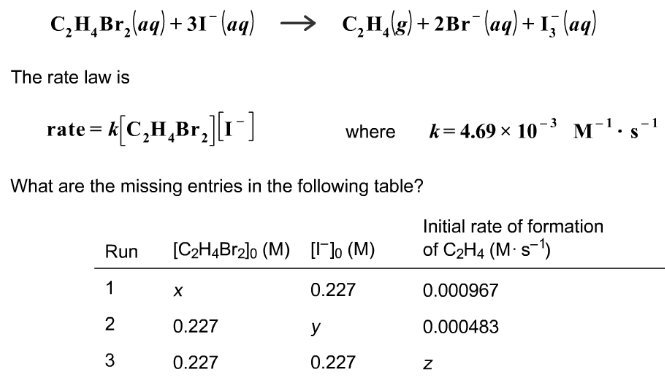# Problem: Consider the reaction described by the following equation.

###### FREE Expert Solution
81% (448 ratings)
###### FREE Expert Solution

For this problem, we are already given the actual rate law complete with the reaction orders and the value of the rate constant. Remember that for rate law, we only care about the reactants so we just disregard the products.

81% (448 ratings)###### Problem Details

Consider the reaction described by the following equation.What scientific concept do you need to know in order to solve this problem?

Our tutors have indicated that to solve this problem you will need to apply the Rate Law concept. You can view video lessons to learn Rate Law. Or if you need more Rate Law practice, you can also practice Rate Law practice problems.

What is the difficulty of this problem?

Our tutors rated the difficulty ofConsider the reaction described by the following equation. ...as medium difficulty.

How long does this problem take to solve?

Our expert Chemistry tutor, Dasha took 9 minutes and 22 seconds to solve this problem. You can follow their steps in the video explanation above.

What professor is this problem relevant for?

Based on our data, we think this problem is relevant for Professor Orgill's class at UNLV.